# Time Series Analysis: Exponential Smoothing Forecasting

The following data (stored in Treasury) represent the three-month Treasury bill rates in the United States from 1991 to 2008:

YearRateYearRate
19915.3820005.82
19923.4320013.40
19933.0020021.61
19944.2520031.01
19955.4920041.37
19965.0120053.15
19975.0620064.73
19984.7820074.36
19994.6420081.37

## A. Plot the data

Step 1

Copy and paste data on excel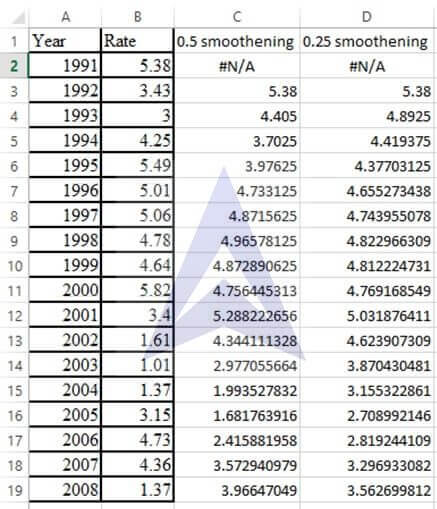Step 2

Plot the line chart of the data by clicking on insert and click on the line chart icon. Select cells A1 to A19 for x axis values and cells B1 to B19 for y axis values.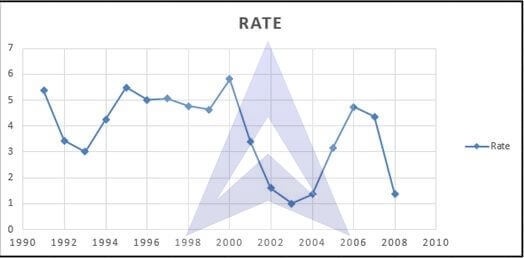## B. Fit a three-year moving average to the data and plot the results

Step 3

Select points the data points on the graph and right click. Click on the option add trend line. A dialogue box will appear select moving average and change the period to 3. Click ok.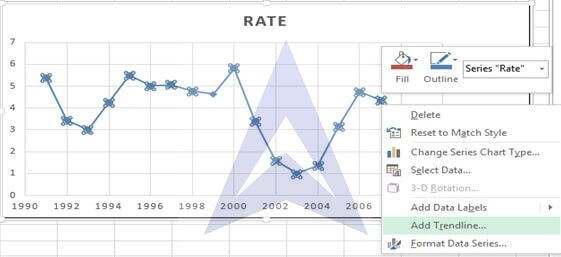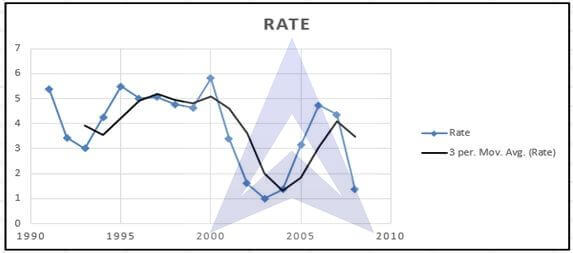## C. Using a smoothing coefficient of W = 0.50, exponentially smooth the series and plot the results

Step 4

Click on data and select data analysis tool pack. A dialogue box opens. Select exponential smoothing from the dialogue box.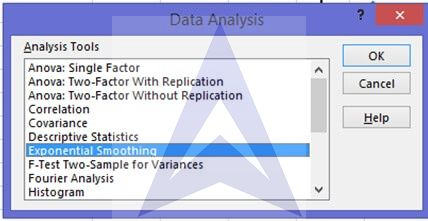Step 5

Select cells B2 to B19 as input range and enter the damping factor as 0.5 (damping factor= 1-smoothing factor (W)) and cell C2 as the starting cell for the output of the smoothing.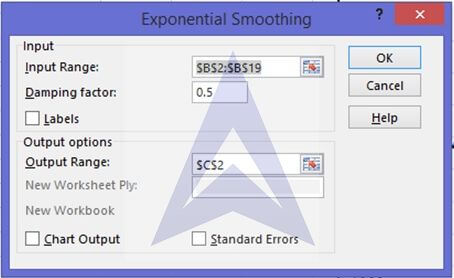You will get the following data.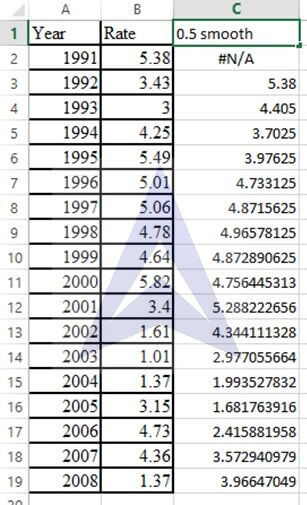Step 6

Right click on the graph. A dialogue box appears click on select data. And click on Add series.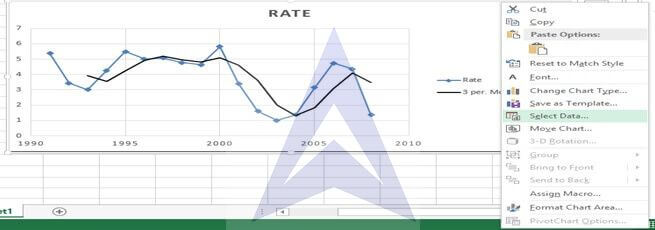The following dialogue box appear. Name the series as 0.5 smooth and for x values select cells A2 to A19 and for y values select cells from C2 to C19. Click ok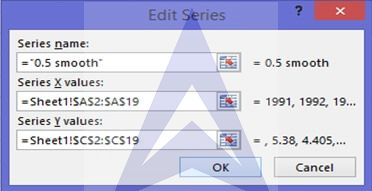The following graphs appears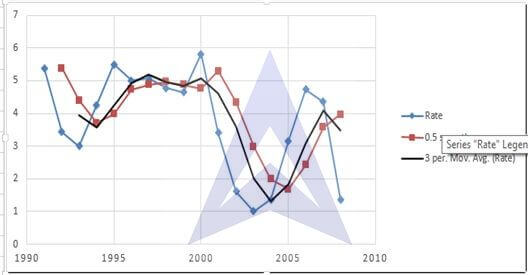## D. What is your exponentially smoothed forecast for 2009?

Step 7

Click on the data points of the graph and right click. Select add trend line. A trend line will appear. Select the trend line and right click. Click on format trend line and select display equation andR2.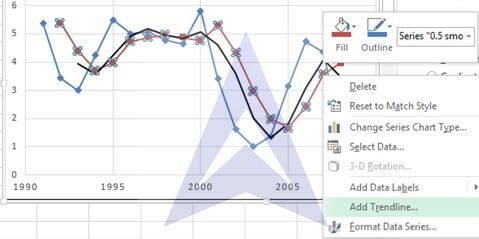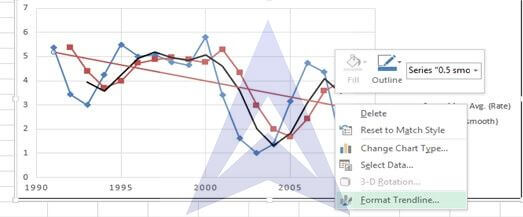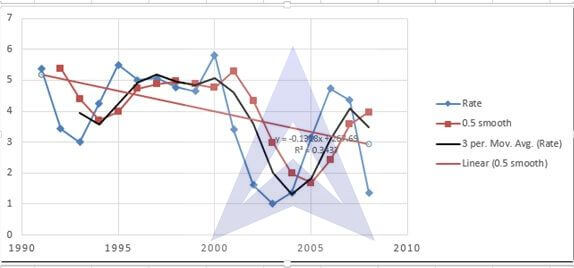```{`
The forecast equation is
Y= -0.1318*x + 5.3128
For x=19 (2009)
Y= -0.1318*19 + 5.3128
= 2.8086
`}```

## E. Repeat (c) and (d), using a smoothing coefficient of W = 0.25

Repeat steps 4-7. Take damping factor as 0.75

After completing the exponential smoothing steps as we did above the following output will be obtained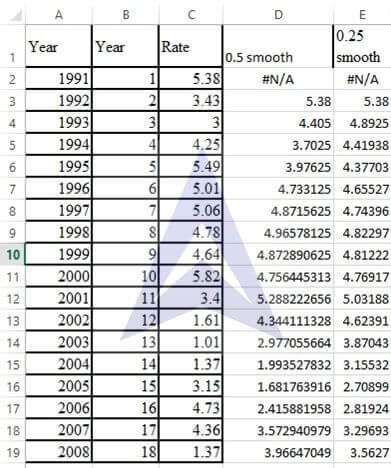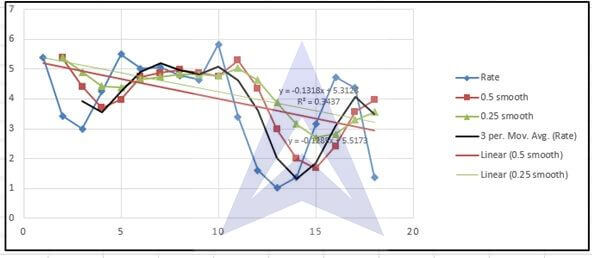```{`
The exponentially smoothed Forecast equation when it is smoothed with W=0.25 is
Y = -0.1285x + 5.5173
The exponential smoothing forecast for 2009 is
Y = -0.1285x + 5.5173
= 3.0758
`}```

## F. Compare the results of (d) and (e)

Comparing the results of D and E we can see that the results with exponential smoothing W=0.5 are closer to the actual data points. It can also be noted that the exponential smoothen trend with lesser W will have lesser peaks and valleys and will be farther from the actual data points when compared with exponential smoothened trend with higher W.

## Excel Econometrics Tutorials

Multiple Regression Analysis

Time Series Analysis

Data Analysis